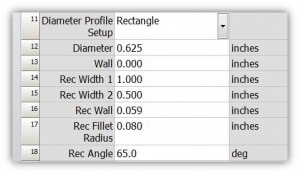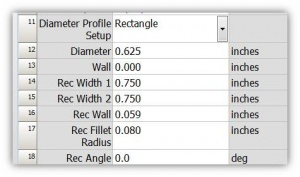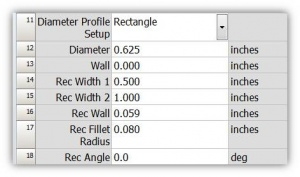# VTube Rectangular Tubes

 As of version 1.82, VTube-STEP and VTube-LASER can draw, calculate, and measure rectangular tube shapes.

## Drawing Rectangular Tube Shapes

 VTube can draw rectangular or square tubing. The dimensional controls are in the Part Setup menu of both STEP and LASER modes:### VTube - Profile Shapes

#### Square TubeSet Width1 and Width2 to the same values to draw square tube.

#### Rectangular - Bending "Easy Way"This profile orientation is referred to as the "easy way" bending in the tubing industry. The reason is because this orientation is easier to bend than a profile rotated 90 degrees to this.

#### Rectangular - Bending "Hard Way"You can switch the values in the Width1 and Width2 to rotate the tube shape into the "hard way" bending method.

### VTube - Profile Rotations

#### Square Tube Rotation

Set the Rectangular Angle to rotate the profile of a square tube relative to the plane of the first bend.

#### Rectangular Tube Rotation

The same rotation feature works for rectangular tubing.

## Extracting Rectangular Shape Centerlines from Models

 VTube can calculate the centerline of rectangular tube solid models. The operator imports the STEP and IGES model, collects the fillet corners of a rectangular shape to calculate the centerline of the rectangular solid model, then calculates the centerline. OUTER DIAMETER SELECTION:Three fillets are selected in each straight's out diameter. This will calculate the centerline. WALL THICKNESS: The wall thickness can also be extracted from the model by selecting one of the inner diameter fillets in any straight. RECTANGULAR PROFILE: If possible, VTube will calculate the rectangular profile widths, fillet radii, and the profile start angle to the first bend plane.

## Measuring Rectangular Tubes

 VTube measures rectangular and square tube shapes with the laser or ball probes. FILLET EDGE DETECTION:The laser scanning feature includes edge detection in order to remove the fillet from the plane calculations. In this image, see how VTube detects edge fillets and removes them automatically from the calculation of the planes. TRUE CENTERLINE LOCATION: The process and logic automatically calculates the location of the centerline from the true surface data. The nominal width data is not needed to find the centerline. OVAL TUBE with FLAT SIDES: VTube can also measure rectangular oval tube shapes with large radius edges using the ball probe. (This profile shape of tube is often used on exercise equipement like [Cybex machines], and in custom exhaust like SpinTech Performance.)